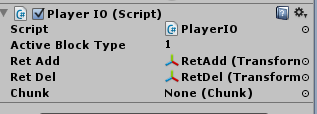# Voxel C# source problems.

So, I’m working on a small project to make a browser game that’s like Minecraft. I’m working along side YouTube instruction videos and some of the project is turning out good, but I’m currently facing two problems.

First, The z positioning walls will not draw. Here’s my code:

``````using UnityEngine;
using System.Collections;
using System.Collections.Generic;

[RequireComponent (typeof(MeshFilter))]
[RequireComponent (typeof(MeshCollider))]
public class Chunk : MonoBehaviour {
public int width = 20;
public byte [,,] map;

protected Mesh mesh;

protected List<Vector3> verts = new List<Vector3>();
protected List<int> tris = new List<int>();
protected List<Vector2> uv = new List<Vector2>();

protected MeshCollider meshCollider;

// Use this for initialization
void Start () {
meshCollider = GetComponent<MeshCollider>();
map = new byte[width, width, width];
for (int x = 0; x < width; x++)
{
for (int z = 0; z < width; z++)
{
map[x, 0, z] = 1;
map[x, 1, z] = (byte)Mathf.RoundToInt(Random.value);
}
}

mesh = new Mesh();
GetComponent<MeshFilter>().mesh = mesh;

Regenerate();
}

// Update is called once per frame
void Update () {

}

public void DrawBrick(int x, int y, int z, byte block)
{
Vector3 start = new Vector3(x,y,z);
Vector3 offset1, offset2;

if(IsTransparent(x, y - 1, z))
{
offset1 = Vector3.left;
offset2 = Vector3.back;
DrawFace(start + Vector3.right, offset1, offset2, block);
}
if(IsTransparent(x, y + 1, z))
{
offset1 = Vector3.right;
offset2 = Vector3.back;
DrawFace(start + Vector3.up, offset1, offset2, block);
}
if(IsTransparent(x - 1, y , z))
{
offset1 = Vector3.up;
offset2 = Vector3.back;
DrawFace(start, offset1, offset2, block);
}
if(IsTransparent(x + 1, y, z))
{
offset1 = Vector3.down;
offset2 = Vector3.back;
DrawFace(start + Vector3.left + Vector3.up, offset1, offset2, block);
}

if(IsTransparent(x, y, z - 1))
{
offset1 = Vector3.right;
offset2 = Vector3.up;
DrawFace(start + Vector3.forward, offset1, offset2, block);
}

if(IsTransparent(x, y, z + 1))
{
offset1 = Vector3.left;
offset2 = Vector3.down;
DrawFace(start + Vector3.up + Vector3.back, offset1, offset2, block);
}

}
public void DrawFace(Vector3 start, Vector3 offset1, Vector3 offset2, byte block)
{
int index = verts.Count;

}

public bool IsTransparent(int x, int y, int z)
{
if ((x < 0) || (y < 0) || (z < 0) || (x >= width) || (y >= width) || (z >=width)) return true;
return map[x,y,z] == 0;
}

public void Regenerate(){
verts.Clear();
tris.Clear();
uv.Clear();
mesh.triangles = tris.ToArray ();
for (int x = 0; x < width; x++)
{
for (int y = 0; y <width; y++)
{
for (int z = 0; z < width; z++)
{
byte block = map[x,y,z];
if (block == 0) continue;

DrawBrick(x, y, z, block);
}
}
}

mesh.vertices = verts.ToArray();
mesh.triangles = tris.ToArray();
mesh.RecalculateNormals();

meshCollider.sharedMesh = null;
meshCollider.sharedMesh = mesh;
}
}
``````

It’s a C# script named ‘Chunk.’ The blocks generate, I can walk on them, but some of the walls will not generate. What directions do I need to set within the z - 1 and z + 1 areas?

Second, I made a file named ‘PlayerIO’ and I have to add a Chunk file (a C# one) to make the rest of it work with the tutorial. I’m able to add everything, but it won’t let me add my chunk file! Here’s the image:When I drag the Chunk C# file into the Chunk section, the ‘No Access’ sort of cursor popsup, and it won’t let me add it.

Does anyone know a solution to these problems? Thank you!

Hello,

When referencing a object in a script, such as your variable `chunk` of type `Chunk` in `PlayerIO`, you need to reference an instance of that object, not the script (assembly) containing that object’s (class’s) definition. One example would be a prefab containing only an object with the `Chunk` script attached.

Hope this helps,
Benproductions1